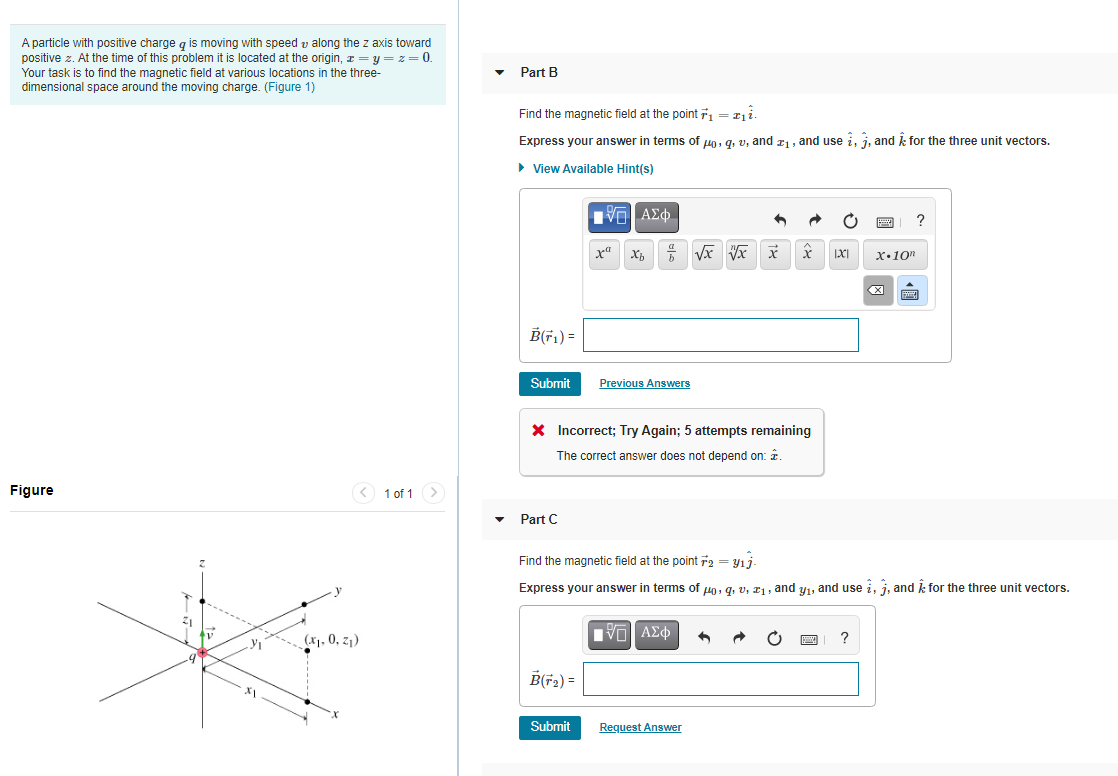# Question Please solve this : A particle with positive charge q is moving with speed v along the z axis toward positive z. At the time of this problem it is located at the origin, 1=y=z=0. Your task is to find the magnetic field at various locations in the three- dimensional space around the moving charge. (Figure 1) Part B Find the magnetic field at the point ří = I1i. Express your answer in terms of p, q, v, and 11, and use i, j, and for the three unit vectors. View Available Hint(s) IVO AED 6VxVx X x XI X.101 BGF)- Submit Previous Answers X Incorrect; Try Again; 5 attempts remaining The correct answer does not depend on: i Figure < 1 of 1 Part C Find the magnetic field at the point F2 = y1j Express your answer in terms of Ho, q, v, 11, and yı, and use i, j, and k for the three unit vectors. (x1.0, 21) IVO ACO yi ? .4 B(+2) = * Submit Request AnswerPlease solve this :